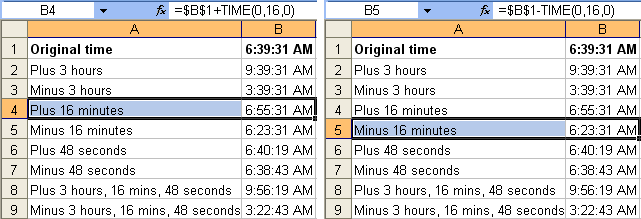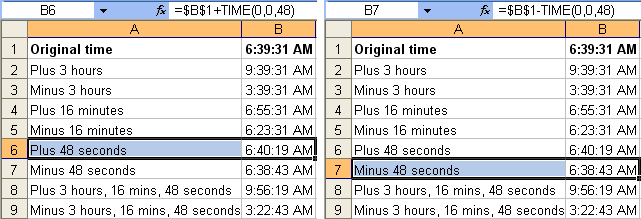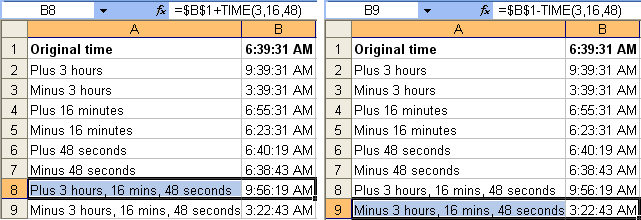EVBA’s Tutorials For Excel: Adding and Subtracting Time in Hours Minutes and Seconds - KING OF EXCEL

## Friday, December 20, 2019

EVBA’s Tutorials For Excel: Adding and Subtracting Time in Hours Minutes and Seconds
Formula examples for hours, minutes, and seconds being added or subtracted from time.
Minutes
Example to add 16 minutes: `=\$B\$1+TIME(0,16,0)`
Example to subtract 16 minutes: `=\$B\$1-TIME(0,16,0)`Seconds
Example to add 48 seconds: `=\$B\$1+TIME(0,0,48)`
Example to subtract 48 seconds: `=\$B\$1-TIME(0,0,48)`Combination of Hours, Minutes, and Seconds
Example to add 3 hours, 16 minutes, and 48 seconds: `=\$B\$1+TIME(3,16,48)`
Example to subtract 3 hours, 16 minutes, and 48 seconds: `=\$B\$1-TIME(3,16,48)`#evba #etipfree
📤You download App EVBA.info installed directly on the latest phone here : https://www.evba.info/p/app-evbainfo-setting-for-your-phone.html?m=1